# CBSE Class 2 Maths Worksheet - Footprints Notes | EduRev

## Class 2 : CBSE Class 2 Maths Worksheet - Footprints Notes | EduRev

The document CBSE Class 2 Maths Worksheet - Footprints Notes | EduRev is a part of the Class 2 Course Mathematics (Maths) for Class 2.
All you need of Class 2 at this link: Class 2

Q.1. Label each shape:
(i)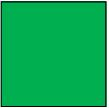Ans.
Square

(ii)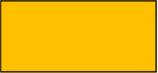Ans.
Rectangle

(iii)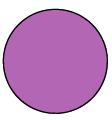Ans.
Circle

(iv)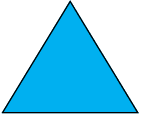Ans.
Triangle

(v)Ans.
Parallelogram

(vi)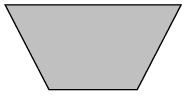Ans.
Trapezoid

(vii)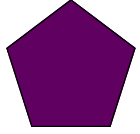Ans.
Pentagon

(viii)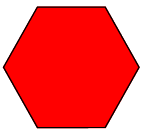Ans.
Hexagon

Q.2. (A) Find the volume of each cube.
(i)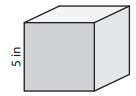Volume =_____

Ans. 125 in3

(ii)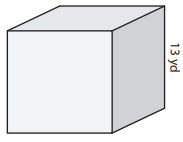Volume = _____

Ans. 2,197 yd3

(iii)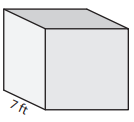Volume = ____
Ans.
343 ft3

(B) Find the volume of each cube from the given side length.
(i) side length = 8 ft
Volume =____

Ans. 512 ft3

(ii) side length = 20 in
Volume = ____
Ans.
8,000 in3

(iii)  side length = 11 in
Volume = ___
Ans.
1,331 in3

(iv) side length = 3 yd
Volume = ___
Ans.
27 yd3

(v) The length of each side of a cubical wooden block is 16 inches. What is the volume of the block?
Ans.
4,096 cubic inches

Q.3. (a) Find the side length of each cube. Round your answer to the nearest tenth.
(i) Volume = 512 in3
side length = ____
Ans.
8 in

(ii) Volume = 6,738 ft3
side length = ____
Ans.
18.9 ft

(iii) Volume = 125 yd3
side length = ____
Ans.
5 yd

(iv)  Volume = 2,197 ft3
side length = ____
Ans.
13 ft

(v) Volume = 44 yd3
side length = ____
Ans.
3.5 yd

(vi) Volume = 3,873 in3
side length = ____
Ans.
15.7 in

(b) A cubical sandbox has a volume of 91,125 cubic inches. What is the side length of the sandbox?
Ans.
45 inches

(c)  What is the side length of a cubical perfume bottle whose volume is 15 cubic inches? Round your answer to the nearest tenth.
Ans.
2.5 inches

Offer running on EduRev: Apply code STAYHOME200 to get INR 200 off on our premium plan EduRev Infinity!

## Mathematics (Maths) for Class 2

53 videos|45 docs|21 tests

,

,

,

,

,

,

,

,

,

,

,

,

,

,

,

,

,

,

,

,

,

;